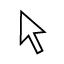# FINDING THE SURFACE AREA OF THE CYLINDER

## Instructions

Introduction   Finding the total area of the surface of a 3-D shape like a cylinder can be very useful.  It can answer questions like, “How much paint do I need to cover the surface?” or “How much wrapping paper do I need to wrap this container?”   There are formulas that you can memorize and use when needed.  However,it is easy to forget a formula that you only use once in a great while.   Is there another way to solve a surface area problem without memorizing the formula?  In this GeoGebra lab you will explore another method for finding surface area.   Step1.  Create and Experiment with a Net Use the MOVE toolto move the Unfold / Fold Net slider.  This will show how the cylinder can be turned into a net. A net is created by imagining that you cut and unfold the 3-D shape into one, flat shape. Using the same slider, the net can be folded back into the original 3-D shape.    Step 2. Find the Surface Area   Suppose that you are given the height (h) of the cylinder and the radius (r) of the top and bottom of the cylinder.  To keep the calculation simple, let’s say that the value of r (the radius of the circle) is 1 unit and the value of height (h) is 2 units.   Once the cylinder is transformed into a net, the problem of finding the surface area is now a problem of finding the area of two shapes.  Which two shapes?   Before continuing, review how you calculate (a) the area of a rectangle, (b) the area of a circle, and (c) the perimeter of a circle. You have enough information to find the area of the two circles, but what about the blue rectangle?  You know the height (h), but what about the width? You need that information to find the area of the rectangle.   Use the Rotate the Top Circle slider to give you a clue to the width of the blue rectangle. Hint:  Watch the dot on the rotating circle.  How many times does the circle rotate in going from one side of the rectangle to the other side?     Now you have enough information to find the area of each piece of the net.  Describe your method for finding the total surface area:   Conclusion   For a cube, the “official” formula is:  surface area = 2 π r2 + 2 π r h But if you forget the formula, you can always use reasoning and a net to help you compute the surface area.